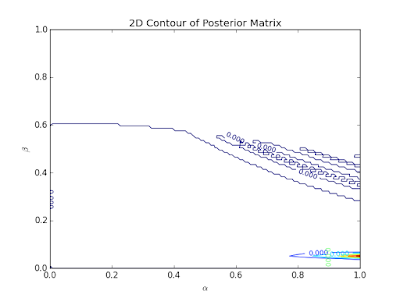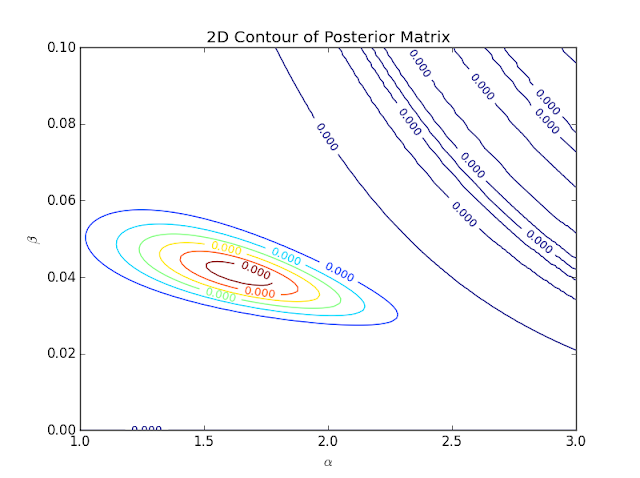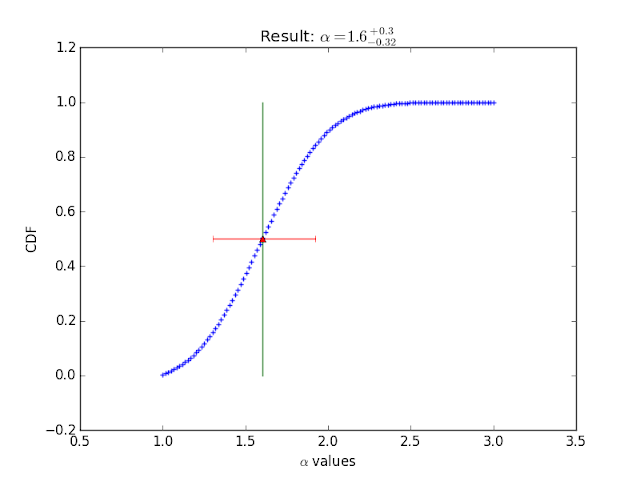## Monday, August 17, 2015

### Astro-Stats : Bayes' Theorem (for Astrophysics), cont.

Now, it is time for us to apply what I explained in the previous post to Python code.  As always, you can follow along on your computer if you have Python installed.  You can copy my code (from here) and paste it into your own file, or you can download my file using the "wget" command in the Terminal. (And don't forget to get the necessary text file mentioned in the previous post.)

I will lead you into this exercise a little differently than I typically do.  I will first show you an unsatisfactory fitting.  This will allow you to see how the process towards finding a satisfactory fit goes sometimes.  After exhibiting the unsatisfactory result, we will learn from the previous results and then run my code again to find the better results.

>>>
>>>execfile('companion_detect.py')
>>>p_posterior(metal_0, metal_1, alphas=np.linspace(0,1,100), betas=np.linspace(0,1,120), plot=True)
>>>

As stated in the previous post, many priors (for each parameter) must be inserted to perform the Grid Search methodology.  When running the code, we have just input 100 different values for the $$\alpha$$ parameter that lie between 0 and 1.  120 numbers between 0 and 1 were chosen as the priors for $$\beta$$.  The contour plot shows the Likelihood for each combination of $$\alpha$$ and $$\beta$$.Figure 1: Contour plot of Likelihoods for the two parameters.  Colors blue to red represent least to largest Likelihoods respectively.

I know what you're thinking... "This is an ugly plot.  Did something go wrong?"  Well, no.  Everything is fine with my code.  This ugliness insinuates that the priors assigned were far from correct.  Notice how the reddish area is barely visible?  This means that values near 1.0 for $$\alpha$$ yield higher Likelihoods than the blue or colorless regions.  The location of the red area also implies that values near 0.1 seem promising for the $$\beta$$ parameter.Figure 2: Terrible looking cumulative distribution function (CDF) plot.  This CDF was suppose to derive from a normal probability distribution function (PDF).  This plot suggests that the PDF was not of a normal distribution.  This is unfortunate primarily because the parameter and its uncertainties were chosen by assuming that the PDF was Gausssian.

If you want to understand the intricate details about how the plot of Figure 2 came about, read my Grad Level Intro... post and the Grad Level Intro... cont. post.  Those posts will also explain why the PDF and CDF are important.  For now, I'll just say that the purpose of Figure 2 is to provide the best fitting parameter.  There are two reasons why I am not satisfied with the result given by Figure 2.  The first reason for my disdain is the hideosity of the plots (and yes, that is a real word).  The second is the non-Gaussian PDF.  Figure 1 and 2 plots suggest that this fitting did not go well and that the unseen PDF is not of a normal distribution although it should be.Figure 3: Atrocious attempt at finding the best fitting value for $$\beta$$.
The disgusting plots of Figures 2 and 3 only confirm the terrible fitting job that was insinuated first by Figure 1.

Well, all is not lost!  As stated previously, the contour plot gave us a clue as to how we can ameliorate the fitting.  Now, let's run the code with different priors.

>>>
>>>execfile('companion_detect.py')
>>>p_posterior(metal_0, metal_1, alphas=np.linspace(0,0.1,100), betas=np.linspace(1,3,120), plot=True)

Now, our $$\alpha$$ priors range from 0 to 0.1 and our $$\beta$$ priors span from 1 to 3.  Let's check out the results!Figure 4: Contour plot for the grid search methodology employed.  The Likelihoods calculated are so small that every number shown seems to be the same because only the first 4 digits are shown.  However, I know that the colors represent the different Likelihoods from blue (least) to red (greatest).
That contour plot looks much more aesthetic than the previous one.  However, the number situation can be very confusing at first glance.Figure 5: Plot of the CDF for the $$\alpha$$ parameter.  The value yielding the best fit was 1.6 for this parameter.Figure 6: Plot of CDF for $$\beta$$ parameter.

There are your results!  Although, I do assume that the results would be more accurate if I were to constrain the range of priors more.

I wanted to show the bad fitting first as a way to convey the detective-like nature of astrophysics researchers.  Researchers, no matter how smart, almost never get the accurate or most satisfactory results on the first try.  This is why one must put on the detective hat and sniff for clues as to what might have gone wrong during the analysis.  I looked for clues in that first contour plot once I noticed how "fishy" it looked.  Figure 1 led me to choosing better priors for my second attempt at the Grid Search.  Moments like that reaffirm my love for plots.  Experiences like that have persuaded me to create many plots as I write complex code.  The graphics can really give a person the ability to see subtle issues that may be visually conspicuous in one's code or methodology that may not be discerned by merely glancing at a bunch of numbers on a computer screen.